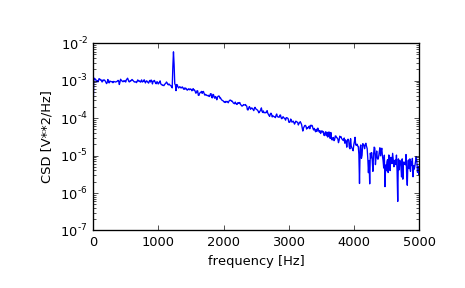# scipy.signal.csd¶

scipy.signal.csd(x, y, fs=1.0, window='hann', nperseg=None, noverlap=None, nfft=None, detrend='constant', return_onesided=True, scaling='density', axis=-1)[source]

Estimate the cross power spectral density, Pxy, using Welch’s method.

Parameters: x : array_like Time series of measurement values y : array_like Time series of measurement values fs : float, optional Sampling frequency of the x and y time series. Defaults to 1.0. window : str or tuple or array_like, optional Desired window to use. See get_window for a list of windows and required parameters. If window is array_like it will be used directly as the window and its length must be nperseg. Defaults to a Hann window. nperseg : int, optional Length of each segment. Defaults to None, but if window is str or tuple, is set to 256, and if window is array_like, is set to the length of the window. noverlap: int, optional Number of points to overlap between segments. If None, noverlap = nperseg // 2. Defaults to None. nfft : int, optional Length of the FFT used, if a zero padded FFT is desired. If None, the FFT length is nperseg. Defaults to None. detrend : str or function or False, optional Specifies how to detrend each segment. If detrend is a string, it is passed as the type argument to the detrend function. If it is a function, it takes a segment and returns a detrended segment. If detrend is False, no detrending is done. Defaults to ‘constant’. return_onesided : bool, optional If True, return a one-sided spectrum for real data. If False return a two-sided spectrum. Note that for complex data, a two-sided spectrum is always returned. scaling : { ‘density’, ‘spectrum’ }, optional Selects between computing the cross spectral density (‘density’) where Pxy has units of V**2/Hz and computing the cross spectrum (‘spectrum’) where Pxy has units of V**2, if x and y are measured in V and fs is measured in Hz. Defaults to ‘density’ axis : int, optional Axis along which the CSD is computed for both inputs; the default is over the last axis (i.e. axis=-1). f : ndarray Array of sample frequencies. Pxy : ndarray Cross spectral density or cross power spectrum of x,y.

periodogram
Simple, optionally modified periodogram
lombscargle
Lomb-Scargle periodogram for unevenly sampled data
welch
Power spectral density by Welch’s method. [Equivalent to csd(x,x)]
coherence
Magnitude squared coherence by Welch’s method.

Notes

By convention, Pxy is computed with the conjugate FFT of X multiplied by the FFT of Y.

If the input series differ in length, the shorter series will be zero-padded to match.

An appropriate amount of overlap will depend on the choice of window and on your requirements. For the default ‘hann’ window an overlap of 50% is a reasonable trade off between accurately estimating the signal power, while not over counting any of the data. Narrower windows may require a larger overlap.

New in version 0.16.0.

References

 [R213] P. Welch, “The use of the fast Fourier transform for the estimation of power spectra: A method based on time averaging over short, modified periodograms”, IEEE Trans. Audio Electroacoust. vol. 15, pp. 70-73, 1967.
 [R214] Rabiner, Lawrence R., and B. Gold. “Theory and Application of Digital Signal Processing” Prentice-Hall, pp. 414-419, 1975

Examples

>>> from scipy import signal
>>> import matplotlib.pyplot as plt


Generate two test signals with some common features.

>>> fs = 10e3
>>> N = 1e5
>>> amp = 20
>>> freq = 1234.0
>>> noise_power = 0.001 * fs / 2
>>> time = np.arange(N) / fs
>>> b, a = signal.butter(2, 0.25, 'low')
>>> x = np.random.normal(scale=np.sqrt(noise_power), size=time.shape)
>>> y = signal.lfilter(b, a, x)
>>> x += amp*np.sin(2*np.pi*freq*time)
>>> y += np.random.normal(scale=0.1*np.sqrt(noise_power), size=time.shape)


Compute and plot the magnitude of the cross spectral density.

>>> f, Pxy = signal.csd(x, y, fs, nperseg=1024)
>>> plt.semilogy(f, np.abs(Pxy))
>>> plt.xlabel('frequency [Hz]')
>>> plt.ylabel('CSD [V**2/Hz]')
>>> plt.show()#### Previous topic

scipy.signal.welch

#### Next topic

scipy.signal.coherence